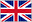# How much vs How many - gramática inglesa

0    10 speciālā zīme

jautājumsatbilde(...) is it?
how much / how many
How much is it?
For (...) people?
how much / how many
For how many people?
(...) tea do you drink every day?
how much / how many
How much tea do you drink every day?
(...) chairs do we need?
how much / how many
How many chairs do we need?
(...) is enough?
how much / how many
How much is enough?
(...) time do you need?
how much / how many
How much time do you need?
(...) drinks did he have?
how much / how many
How many drinks did he have?
(...) water is in the bottle?
how much / how many
How much water is in the bottle?
(...) of you agree?
how much / how many
How many of you agree?
(...) is three times five?
how much / how many
How much is three times five?